# Search Results (5)

View
Selected filters:
• Complex Numbers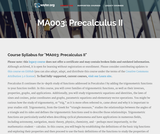Conditions of Use:
قراءة النص المطبوع بخط رفيع
Rating

This course begins by establishing the definitions of the basic trig functions and exploring their properties, and then proceeds to use the basic definitions of the functions to study the properties of their graphs, including domain and range, and to define the inverses of these functions and establish the their properties. Through the language of transformation, the student will explore the ideas of period and amplitude and learn how these graphical differences relate to algebraic changes in the function formulas. The student will also learn to solve equations, prove identities using the trig functions, and study several applications of these functions. Upon successful completion of this course, the student will be able to: measure angles in degrees and radians, and relate them to arc length; solve problems involving right triangles and unit circles using the definitions of the trigonometric functions; solve problems involving non-right triangles; relate the equation of a trigonometric function to its graph; solve trigonometric equations using inverse trig functions; prove trigonometric identities; solve trig equations involving identities; relate coordinates and equations in Polar form to coordinates and equations in Cartesian form; perform operations with vectors and use them to solve problems; relate equations and graphs in Parametric form to equations and graphs in Cartesian form; link graphical, numeric, and symbolic approaches when interpreting situations and analyzing problems; write clear, correct, and complete solutions to mathematical problems using proper mathematical notation and appropriate language; communicate the difference between an exact and an approximate solution and determine which is more appropriate for a given problem. This free course may be completed online at any time. It has been developed through a partnership with the Washington State Board for Community and Technical Colleges; the Saylor Foundation has modified some WSBCTC materials. (Mathematics 003)

نوع المادة:
المقرر التعليمي الكامل
Provider:
The Saylor Foundation
04/16/2012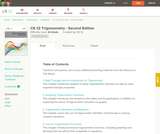Conditions of Use:
يمكن إعادة ترتيب المحتوى ومشاركته
Rating

CK-12 Foundation's Trigonometry FlexBook is an introduction to trigonometry for the high school student. Topics include: Trigonometric Identities & Equations, Circular Functions, and Polar Equations & Complex Numbers.

نوع المادة:
Textbook
Provider:
CK-12 Foundation
Provider Set:
كتاب FlexBook من تصنيف المرحلة من الابتدائية إلى الثانوية
المؤلف:
Fortgang, Art
Hayes, Andrea
Jordan, Lori
Landers, Mara
Meery, Brenda
Ottman, Larry
08/12/2010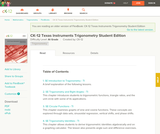Conditions of Use:
يمكن إعادة ترتيب المحتوى ومشاركته
Rating

CK-12's Texas Instruments Trigonometry Student Edition Flexbook is a helpful companion to a trigonometry course, providing students with more ways to understand basic trigonometric concepts through supplementary exercises and explanations.

نوع المادة:
Textbook
Provider:
CK-12 Foundation
Provider Set:
كتاب FlexBook من تصنيف المرحلة من الابتدائية إلى الثانوية
المؤلف:
Jordan, Lori
12/14/2010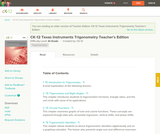Conditions of Use:
يمكن إعادة ترتيب المحتوى ومشاركته
Rating

CK-12's Texas Instruments Trigonometry Teacher's Edition Flexbook is a helpful companion to a trigonometry course, providing students with more ways to understand basic trigonometric concepts through supplementary exercises and explanations.

نوع المادة:
Textbook
Provider:
CK-12 Foundation
Provider Set:
كتاب FlexBook من تصنيف المرحلة من الابتدائية إلى الثانوية
المؤلف:
Jordan, Lori
12/21/2010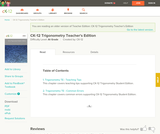Conditions of Use:
يمكن إعادة ترتيب المحتوى ومشاركته
Rating

CK-12 Trigonometry Teacher's Edition provides tips and common errors for teaching CK-12 Trigonometry Student Edition. The solution and assessment guides are available upon request.

نوع المادة:
Textbook
Provider:
CK-12 Foundation
Provider Set:
كتاب FlexBook من تصنيف المرحلة من الابتدائية إلى الثانوية
المؤلف:
Rawley, Eve